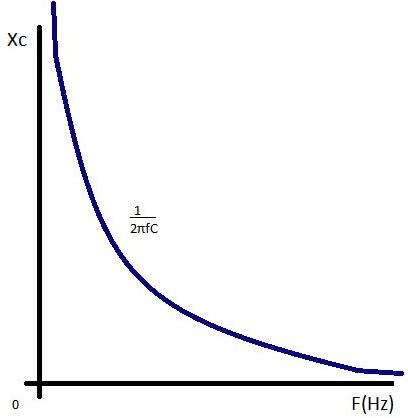Courses

# Test: AC Applied Across Capacitor

## 10 Questions MCQ Test Physics Class 12 | Test: AC Applied Across Capacitor

Description
This mock test of Test: AC Applied Across Capacitor for JEE helps you for every JEE entrance exam. This contains 10 Multiple Choice Questions for JEE Test: AC Applied Across Capacitor (mcq) to study with solutions a complete question bank. The solved questions answers in this Test: AC Applied Across Capacitor quiz give you a good mix of easy questions and tough questions. JEE students definitely take this Test: AC Applied Across Capacitor exercise for a better result in the exam. You can find other Test: AC Applied Across Capacitor extra questions, long questions & short questions for JEE on EduRev as well by searching above.
QUESTION: 1

### Is it possible to use a capacitor of suitable capacitance instead of a choke coil?

Solution:

We can use a capacitor of suitable capacitance as a choke coil because the average power consumed per cycle in an ideal capacitor is zero. Therefore, like a choke coil, a condenser can reduce AC without power dissipation.

QUESTION: 2

### An a.c. voltage source E= 200 √2 sin 100t is connected across a circuit containing an a.c ammeter and a capacitor of capacitance 1μF. The reading of the ammeter is:​

Solution:

equation of alternating voltage , E = 200√2sin(100t)
compare this equation with E0sin(ωt)
so,  E0=200√2 volts
ω= 100 rad/s
now, Erms=E0/√2
= 200√2/√2 = 200 volts .
given, capacitance of capacitor, C = 10-6 F
so, reactance of capacitor,  XC=1/ωC
=1/(10-6x100)
=104
so, the reading of Ammeter, I=Erms/XC
= 200/104 A = 20 × 10-3 A = 20mA
hence, option (b) is correct.

QUESTION: 3

### What is the unit of Capacitive Reactance Xc?​

Solution:

The opposition offered by a capacitor for the flow of A.C is called capacitive reactance.
Xc = 1/wC
it's SI unit is ohm

QUESTION: 4

The average power dissipation in pure capacitive circuit is:

Solution:

As we know,
Average power in ac circuit is given by P=Vrms​irms​cosϕ
For pure capacitive circuit ϕ=90o so P=0

QUESTION: 5

What is the natural frequency of an LC oscillator?

Solution:
QUESTION: 6

What is the average power consumed/cycle in ideal capacitor.​

Solution:

Pav=(V0i0/2) cosθ
In ideal capacitor θ= -π2
Cosθ=Cosθ(-π/2)=0
Therefore
Pav=0

QUESTION: 7

Capacitive reactance of the capacitor depends upon

Solution:

Capacitive reactance (symbol XC) is a measure of a capacitor’s opposition to AC (alternating current). Like resistance it is measured in ohms, but reactance is more complex than resistance because its value depends on the frequency (f) of the signal passing through the capacitor. Reactance is also inversely proportional to the value of capacitance (C), i.e. the value of XC at any frequency will be less in larger capacitors than in smaller ones. All capacitors have infinitely high values of reactance at 0Hz, but in large capacitors, the reactance falls to a low level at much lower frequencies than in smaller capacitors. Hence, larger capacitors are preferred in low frequency applications.QUESTION: 8

he impedance of a 10 microfarad capacitor for 50 Hz ac is:

Solution:

Impedance(XC)=1/ωC
=1/ 2πf λC
=1/2πx50x10x10-6
=10-6/1000π
XC=103/π=(1000/π) Ω

QUESTION: 9

In a ac circuit with capacitance, the current

Solution:

Voltage lags current by 90° in a pure capacitive circuit. In a pure capacitive circuit, the instantaneous power may be positive or negative. As with the simple inductor circuit, the 90-degree phase shift between voltage and current results in a power wave that alternates equally between positive and negative.

QUESTION: 10

What is the capacitive reactance of 6 x 10-6 F capacitor for frequency of 106 Hz?​

Solution: